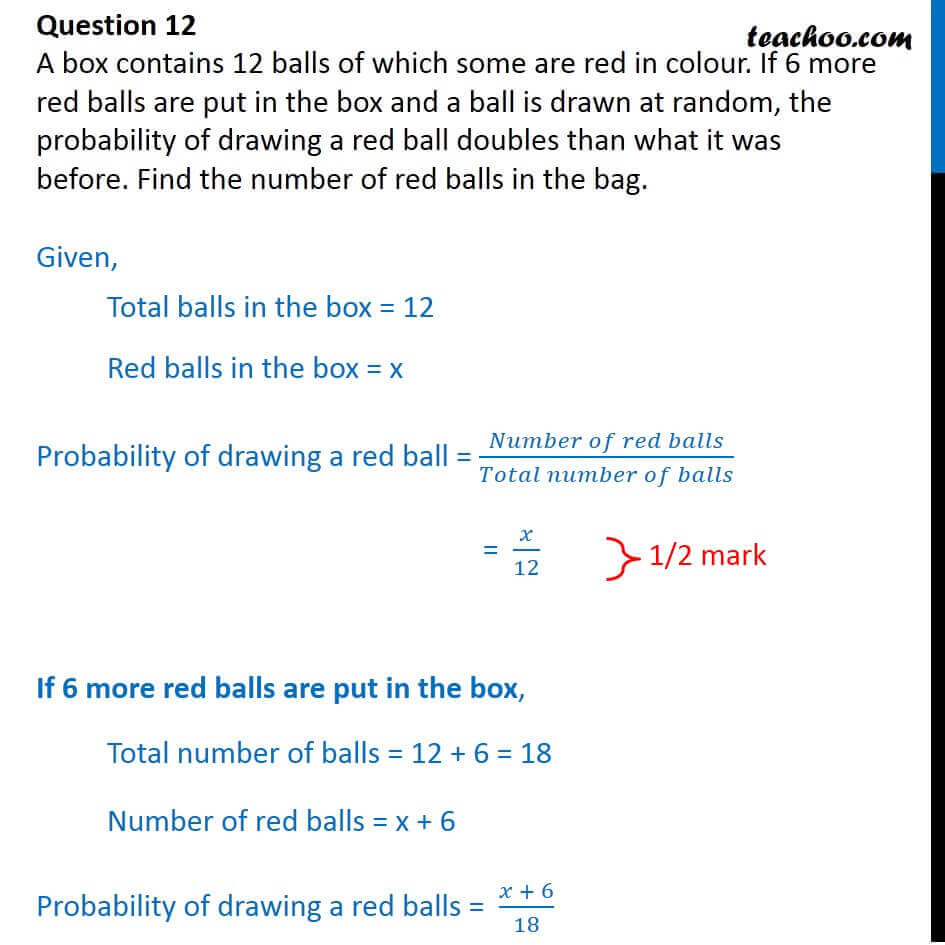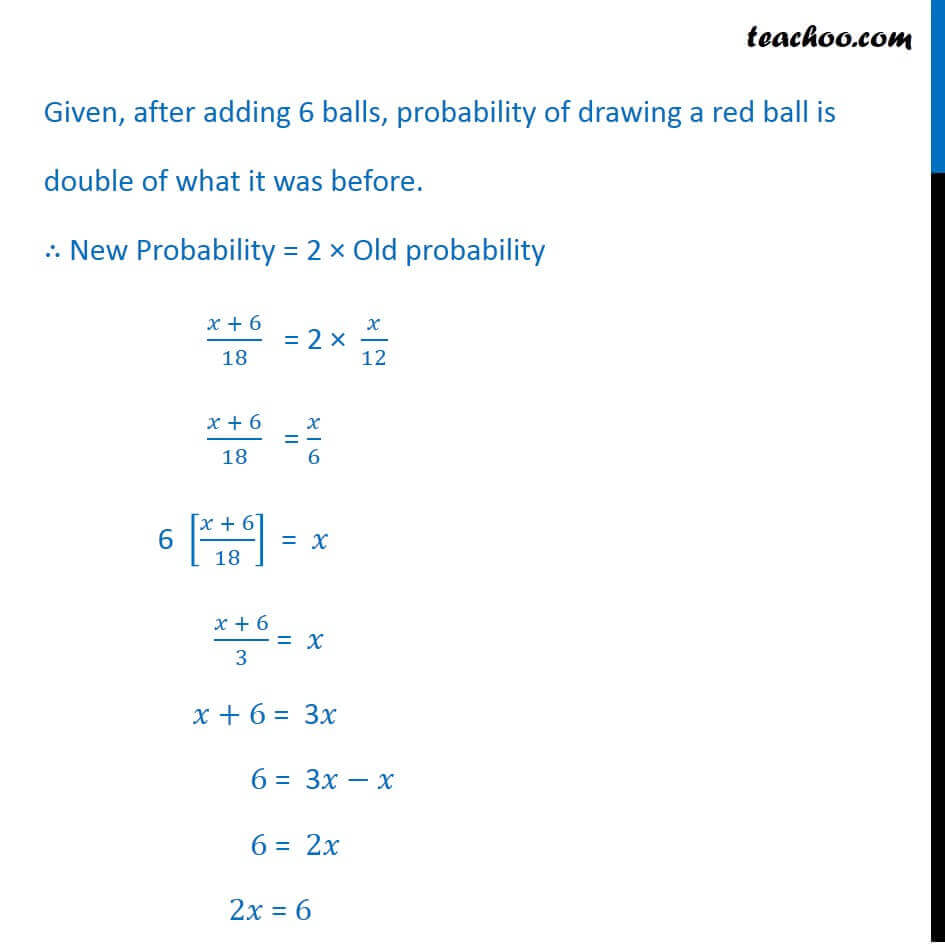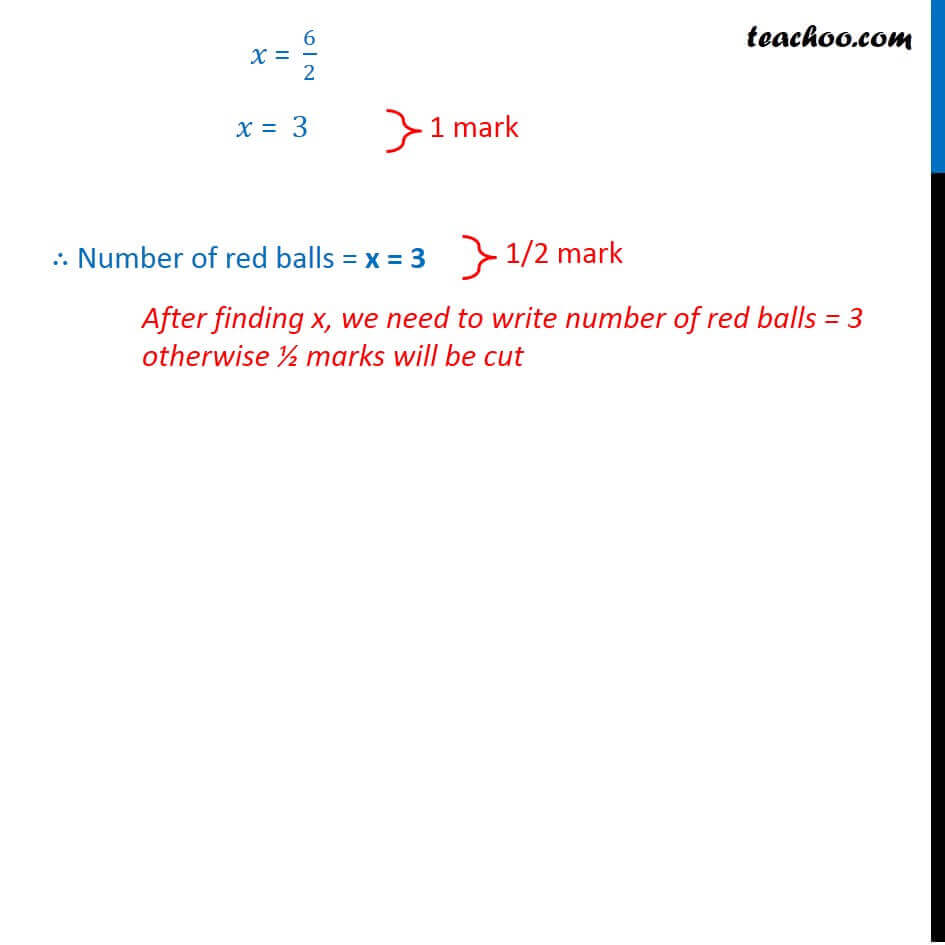CBSE Class 10 Sample Paper for 2018 Boards

Class 10
Solutions of Sample Papers for Class 10 Boards

### A box contains 12 balls of which some are red in colour. If 6 more red balls are put in the box and a ball is drawn at random, the probability of drawing a red ball doubles than what it was before. Find the number of red balls in the bag.

This is a question of CBSE Sample Paper - Class 10 - 2017/18.Get live Maths 1-on-1 Classs - Class 6 to 12

### Transcript

Question 12 A box contains 12 balls of which some are red in colour. If 6 more red balls are put in the box and a ball is drawn at random, the probability of drawing a red ball doubles than what it was before. Find the number of red balls in the bag.Given, Total balls in the box = 12 Red balls in the box = x Probability of drawing a red ball = (𝑁𝑢𝑚𝑏𝑒𝑟 𝑜𝑓 𝑟𝑒𝑑 𝑏𝑎𝑙𝑙𝑠)/(𝑇𝑜𝑡𝑎𝑙 𝑛𝑢𝑚𝑏𝑒𝑟 𝑜𝑓 𝑏𝑎𝑙𝑙𝑠) = 𝑥/12 If 6 more red balls are put in the box, Total number of balls = 12 + 6 = 18 Number of red balls = x + 6 Probability of drawing a red balls = (𝑥 + 6)/18 Given, after adding 6 balls, probability of drawing a red ball is double of what it was before. ∴ New Probability = 2 × Old probability (𝑥 + 6)/18 = 2 × 𝑥/12 (𝑥 + 6)/18 = 𝑥/6 6 [(𝑥 + 6)/18] = 𝑥 (𝑥 + 6)/3 = 𝑥 𝑥+6 = 3𝑥 6 = 3𝑥−𝑥 6 = 2𝑥 2𝑥 = 6 𝑥" = " 6/2 𝑥 = 3 ∴ Number of red balls = x = 3